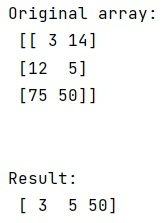# How to select one element in each row of a NumPy array by column indices?

Given a NumPy array, we have to select one element in each row from it by column indices.
Submitted by Pranit Sharma, on March 12, 2023

## NumPy Array | Selecting one element in each row by column indices

Suppose that we are given a numpy array and an array of indices to be selected and we need to select one element in each row of this array using the array of indices.

For this purpose, we can choose from the given array using numpy.choose() which constructs an array from an index array and a set of arrays to choose from. However, we may first need to transpose the input array to match the dimensions.

Let us understand with the help of an example,

## Python code to select one element in each row of a NumPy array by column indices

```# Import numpy
import numpy as np

# Creating a numpy array
arr = np.array([[ 3, 14],[12,  5],[75, 50]])

# Display Original array
print("Original array:\n",arr,"\n\n")

# Array of indices
id_arr = [0, 1, 1]

# Using np.choose to select elements of input array
res = np.choose(id_arr, arr.T)

# Display result
print("Result:\n",res)
```

### OutputLanguages: » C » C++ » C++ STL » Java » Data Structure » C#.Net » Android » Kotlin » SQL
Web Technologies: » PHP » Python » JavaScript » CSS » Ajax » Node.js » Web programming/HTML
Solved programs: » C » C++ » DS » Java » C#
Aptitude que. & ans.: » C » C++ » Java » DBMS
Interview que. & ans.: » C » Embedded C » Java » SEO » HR
CS Subjects: » CS Basics » O.S. » Networks » DBMS » Embedded Systems » Cloud Computing
» Machine learning » CS Organizations » Linux » DOS
More: » Articles » Puzzles » News/Updates